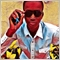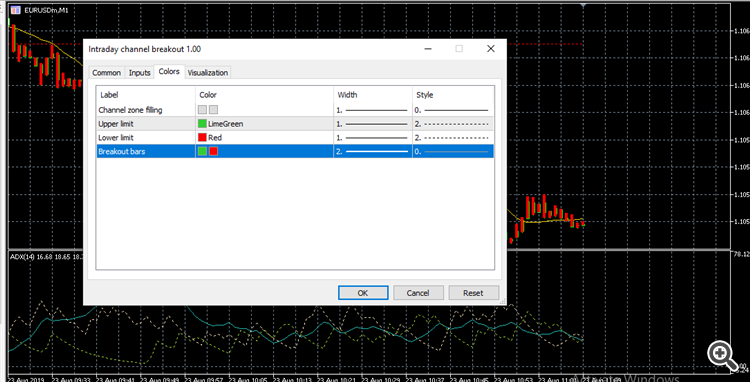# Need Help for some modification11311

Hello.. am creating an EA based on a custom indicator... the problem is filtering the buy and sell signal

after creating the EA to open buy on buffer ''0'' and sell on buffer '1' but the EA will open only sell orders on a buy/sell signal (all signals)

after trying some modifications for 2 days, and some help from some programmers it will open both a buy and sell at once

am sure the problem is from the indicator coding

suggest sell buffer coding separately and buy buffer coding separately

Thanks

```SetIndexBuffer(0,histou);SetIndexStyle(0,ShowBars ? DRAW_HISTOGRAM : DRAW_NONE);
SetIndexBuffer(1,histod);SetIndexStyle(1,ShowBars ? DRAW_HISTOGRAM : DRAW_NONE); ```
```//+------------------------------------------------------------------+
//|                                                 Channel Breakout |
//|                                       Copyright 2019, Abu Saidu. |
//+------------------------------------------------------------------+
//------------------------------------------------------------------
#property version     "1.00"
//------------------------------------------------------------------
#property indicator_chart_window
#property indicator_buffers 6
#property indicator_color1  clrBlue
#property indicator_color2  clrYellow
#property indicator_color3  clrLimeGreen
#property indicator_color4  clrRed
#property indicator_color5  clrGainsboro
#property indicator_color6  clrGainsboro
#property indicator_style3  STYLE_DOT
#property indicator_style4  STYLE_DOT
#property indicator_width1  2
#property indicator_width2  2
#property strict

//---
//
input string    inpStartTime = "00:00";      // Start time
input string    inpEndTime   = "03:59";      // Ending time
input bool      ShowBars     = true;         // Show Breakout bars?

//
//---
//
double  fillu[],filld[],limu[],limd[],histou[],histod[],histoc[];

//------------------------------------------------------------------
//
//------------------------------------------------------------------
//
//
//
//
//

int OnInit()
{
IndicatorBuffers(7);
SetIndexBuffer(0,histou);SetIndexStyle(0,ShowBars ? DRAW_HISTOGRAM : DRAW_NONE);
SetIndexBuffer(1,histod);SetIndexStyle(1,ShowBars ? DRAW_HISTOGRAM : DRAW_NONE);
SetIndexBuffer(2,limu);  SetIndexStyle(2,DRAW_LINE);
SetIndexBuffer(3,limd);  SetIndexStyle(3,DRAW_LINE);
SetIndexBuffer(4,fillu); SetIndexStyle(4,DRAW_LINE);
SetIndexBuffer(5,filld); SetIndexStyle(5,DRAW_LINE);
SetIndexBuffer(6,histoc);

//
//---
//
if (_Period>=PERIOD_D1)
{
Alert("Indicator can work on time frames less than daily only");  return(INIT_FAILED);
}
IndicatorShortName("Channel "+inpStartTime+" "+inpEndTime+" breakout");
return(INIT_SUCCEEDED);
}
void OnDeinit(const int reason) { }

//------------------------------------------------------------------
//
//------------------------------------------------------------------
//
//
//
//
//

int OnCalculate(const int rates_total,
const int prev_calculated,
const datetime& time[],
const double& open[],
const double& high[],
const double& low[],
const double& close[],
const long& tick_volume[],
const long& volume[],
{
int i,counted_bars = prev_calculated;
if(counted_bars < 0) return(-1);
if(counted_bars > 0) counted_bars--;
int limit=MathMin(rates_total-counted_bars,rates_total-1);

//
//
//
//
//

int _secondsStart = (int)StringToTime("1970.01.01 "+inpStartTime);
int _secondsEnd   = (int)StringToTime("1970.01.01 "+inpEndTime);
for (i=limit;i>=0; i--)
{
datetime _startTime = StringToTime(TimeToString(Time[i],TIME_DATE))+_secondsStart;
datetime _endTime   = StringToTime(TimeToString(Time[i],TIME_DATE))+_secondsEnd;
double max = ((i<Bars-1) ? limu[i+1] : High[i]), min = ((i<Bars-1) ? limd[i+1] : Low[i]);
if (_startTime<= Time[i] && _endTime>=Time[i])
{
max = High[i];
min =  Low[i];
for (int k=1; i+k>=0 && Time[i+k]>=_startTime; k++)
{
max = fmax(max,High[i+k]);
min = fmin(min,Low[i+k]);
}
}
limu[i] = max;
limd[i] = min;

if (_startTime<=Time[i] && _endTime>=Time[i])
{
fillu[i]  = max;
filld[i]  = min;
histou[i] = EMPTY_VALUE;
histod[i] = EMPTY_VALUE;
}
else
{
fillu[i]  = (limu[i]+limd[i])*0.5;
filld[i]  = (limu[i]+limd[i])*0.5;
histoc[i] = (i<Bars-1) ? (Close[i]>limu[i]) ? 1 : (Close[i]<limd[i]) ? -1 : (Close[i]<limu[i] && Close[i]>limd[i]) ? 0 : histoc[i+1] : 0;
if (histoc[i] == 1) { histou[i] = High[i]; histod[i] = Low[i]; }
if (histoc[i] ==-1) { histod[i] = High[i]; histou[i] = Low[i]; }
}
}
return(rates_total);
}```25828

Abubakar Saidu: after creating the EA to open buy on buffer ''0'' and sell on buffer '1'
1. Why did you post your MT4 question in the Root / MT5 EA section instead of the MQL4 section, (bottom of the Root page?)
General rules and best pratices of the Forum. - General - MQL5 programming forum
Next time post in the correct place. The moderators will likely move this thread there soon.

2. `if (_startTime<= Time[i] && _endTime>=Time[i])`
Note: your inputs are 0:00 … 3:59 and with the equals your code runs from 0:00:00 … 3:59:00. It does not run on the last minute. By dropping the trailing equals and using 04:00, your code will run from 0:00:00 … 3:59:59 which is what you really want.

3. ```         if (_startTime<=Time[i] && _endTime>=Time[i])
{
fillu[i]  = max;
filld[i]  = min;
histou[i] = EMPTY_VALUE;
histod[i] = EMPTY_VALUE;
```
During your proper times you don't fill in buffers 0/1.
```           if (histoc[i] == 1) { histou[i] = High[i]; histod[i] = Low[i]; }
if (histoc[i] ==-1) { histod[i] = High[i]; histou[i] = Low[i]; }
```
Outside of those times you do fill in the historgrams (blue or yellow.)11311

William Roeder:
1. Why did you post your MT4 question in the Root / MT5 EA section instead of the MQL4 section, (bottom of the Root page?)
General rules and best pratices of the Forum. - General - MQL5 programming forum
Next time post in the correct place. The moderators will likely move this thread there soon.

2. Note: your inputs are 0:00 … 3:59 and with the equals your code runs from 0:00:00 … 3:59:00. It does not run on the last minute. By dropping the trailing equals and using 04:00, your code will run from 0:00:00 … 3:59:59 which is what you really want.

3. During your proper times you don't fill in buffers 0/1.
Outside of those times you do fill in the historgrams (blue or yellow.)

i still don't understand
```histou[i] = EMPTY_VALUE;
histod[i] = EMPTY_VALUE;
```

i changed the EMPTY_VALUE to Buffer_0/Buffer_1

but still nothing but an ERROR

this is the mt5 indicator... it was converted to mq4```//------------------------------------------------------------------
#property version     "1.00"
//------------------------------------------------------------------
#property indicator_chart_window
#property indicator_buffers 7
#property indicator_plots   4
#property indicator_label1  "Channel zone filling"
#property indicator_type1   DRAW_FILLING
#property indicator_color1  clrGainsboro,clrGainsboro
#property indicator_label2  "Upper limit"
#property indicator_type2   DRAW_LINE
#property indicator_color2  clrLimeGreen
#property indicator_style2  STYLE_DOT
#property indicator_label3  "Lower limit"
#property indicator_type3   DRAW_LINE
#property indicator_color3  clrRed
#property indicator_style3  STYLE_DOT
#property indicator_label4  "Breakout bars"
#property indicator_type4   DRAW_COLOR_HISTOGRAM2
#property indicator_color4  clrLimeGreen,clrRed
#property indicator_width4  2
//
//---
//
input string    inpStartTime = "00:00"; // Start time
input string    inpEndTime   = "03:59"; // Ending time
//
//---
//
double  fillu[],filld[],limu[],limd[],histou[],histod[],histoc[];

//------------------------------------------------------------------
//
//------------------------------------------------------------------
//
//
//
//
//

int OnInit()
{
SetIndexBuffer(0,fillu ,INDICATOR_DATA);
SetIndexBuffer(1,filld ,INDICATOR_DATA);
SetIndexBuffer(2,limu  ,INDICATOR_DATA);
SetIndexBuffer(3,limd  ,INDICATOR_DATA);
SetIndexBuffer(4,histou,INDICATOR_DATA);
SetIndexBuffer(5,histod,INDICATOR_DATA);
SetIndexBuffer(6,histoc,INDICATOR_COLOR_INDEX);
//
//---
//
if (_Period>=PERIOD_D1)
{
Alert("Indicator can work on time frames less than daily only");  return(INIT_FAILED);
}
IndicatorSetString(INDICATOR_SHORTNAME,"Channel "+inpStartTime+" "+inpEndTime+" breakout");
return(INIT_SUCCEEDED);
}
void OnDeinit(const int reason) { }

//------------------------------------------------------------------
//
//------------------------------------------------------------------
//
//
//
//
//

int OnCalculate(const int rates_total,
const int prev_calculated,
const datetime& time[],
const double& open[],
const double& high[],
const double& low[],
const double& close[],
const long& tick_volume[],
const long& volume[],
{
if (Bars(_Symbol,_Period)<rates_total) return(-1);
int _secondsStart = (int)StringToTime("1970.01.01 "+inpStartTime);
int _secondsEnd   = (int)StringToTime("1970.01.01 "+inpEndTime);
int i=(int)MathMax(prev_calculated-1,0); for (; i<rates_total  && !_StopFlag; i++)
{
datetime _startTime = StringToTime(TimeToString(time[i],TIME_DATE))+_secondsStart;
datetime _endTime   = StringToTime(TimeToString(time[i],TIME_DATE))+_secondsEnd;
double max = ((i>0) ? limu[i-1] : high[i]), min = ((i>0) ? limd[i-1] : low[i]);
if (_startTime<= time[i] && _endTime>=time[i])
{
max = high[i];
min = low[i];
for (int k=1; i-k>=0 && time[i-k]>=_startTime; k++)
{
max = MathMax(max,high[i-k]);
min = MathMin(min,low[i-k]);
}
}
limu[i] = max;
limd[i] = min;
if (_startTime<=time[i] && _endTime>=time[i])
{
fillu[i] = max;
filld[i] = min;
histou[i] = EMPTY_VALUE;
histod[i] = EMPTY_VALUE;
}
else
{
fillu[i]  = (limu[i]+limd[i])/2.0;
filld[i]  = (limu[i]+limd[i])/2.0;
histou[i] = (close[i]>limu[i] || close[i]<limd[i]) ? high[i] : EMPTY_VALUE;
histod[i] = (close[i]>limu[i] || close[i]<limd[i]) ? low[i]  : EMPTY_VALUE;
histoc[i] = (close[i]>limu[i]) ? 0 : 1;
}
}
return(i);
}
```